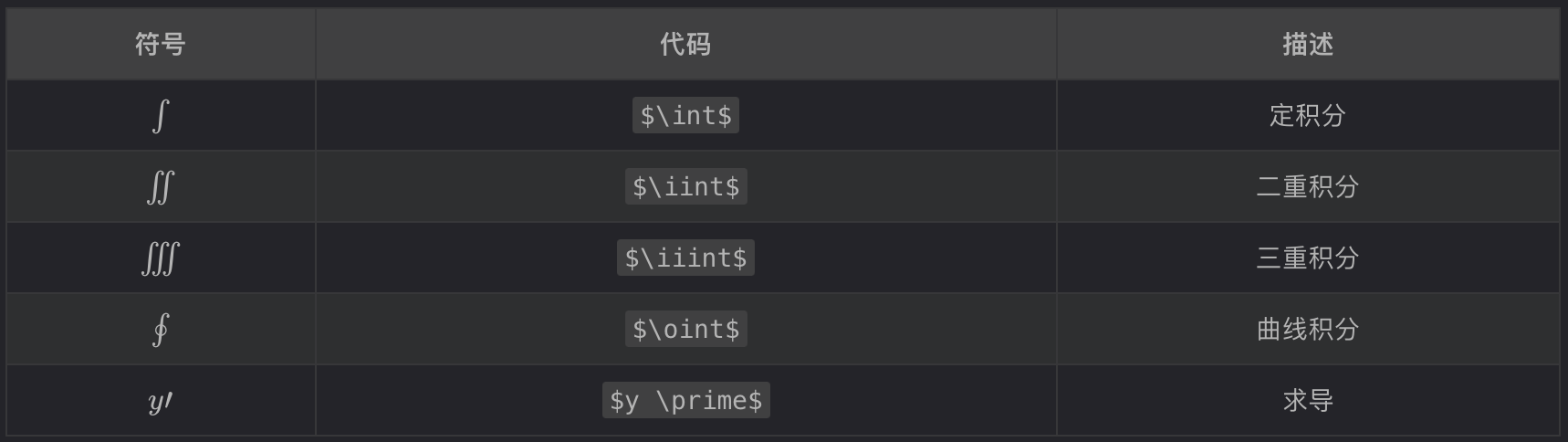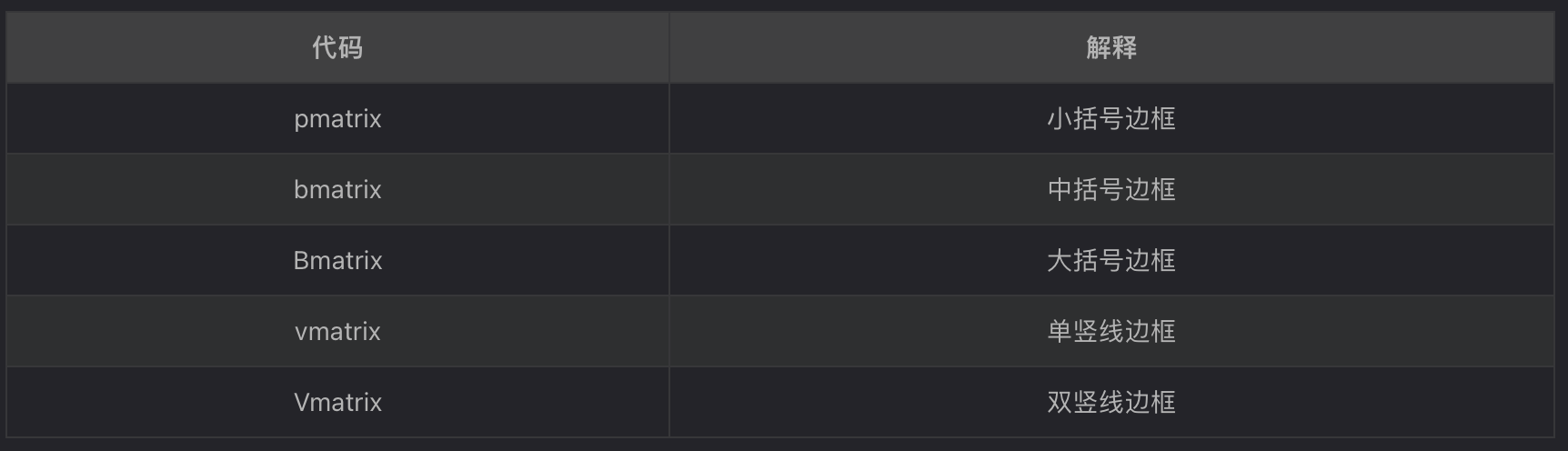## 1. markdown数学公式

markdown数学公式：

• 行内公式： $数学公式$
• 行间公式：$$数学公式$$

1) 上下标

^表示上标， _表示下标，如果上标或下标内容多于一个字符，则使用{}括起来。

$(x^2 + x^2 )^{x^y}+ x_1^2= y_1 - y_2^{x_1^2-y_1^2}$


ps: 这里说一点，在平时中我们完全有两个^表示上标，两个~表示下标，个人感觉这种在不涉及复杂的数学公式单纯表示某个变量或未知数时更方便，相信大家也知道。

2) 分数

$\frac{1+x}{y-1}$ 或 $x \over x+y$


3) 开方

 $\sqrt{4}$ 或 $\sqrt{9}$


4) 括号

• ()[]直接写就行，而 {} 则需要转义（转义：需要左括号前加\和右括号前加\）

$f(x, y) = x^2 + y^2, x \epsilon [0, 100], y \epsilon \lbrace {1,2,3} \rbrace$


• 长括号，需要左括号前加\left和右括号前加\right，（此大括号非彼大括号）

$(\sqrt{1 \over 2})^2$加大括号后 $\left(\sqrt{1 \over 2}\right)^2$


• \left\right必须成对出现，对于不显示的一边可以使用.代替。

$\frac{du}{dx} \mid _{x=0}$ 加大后 $\left. \frac{du}{dx} \right\| _{x=0}$


$\frac{du}{dx} \mid _{x=0}$ 变成 $\left. \frac{du}{dx} \right| _{x=0}$

• 大括号用\begin{cases}表示开始，用\end{cases}表示结束，中间\\来换行 例如：
$f(x,y)=\begin{cases} x^2+y^2=1 \\\ x-y = 0 \end{cases}$


5） 向量 公式\vec{a}

$\vec d \cdot \vec b = 1$


6) 定积分

符号：$\int$，示例公式：$\int_0^1x^2dx$


7) 正负无穷

正无穷：其表达式为$+\infty$



8) 极限

$\lim_{n\rightarrow+\infty}\frac{1}{n}$


ps: 毕竟电脑不能完美替代手写，虽然手写一直放在lim下面

9) 累加累乘

累加$\sum_1^n$



10) 省略号

$f(x_1,x_2,\ldots,x_n) = \left({1 \over x_1}\right)^2+\left({1 \over x_2}\right)^2+\cdots+\left({1 \over x_n}\right)^2$


11） 数学符号12) 三角函数13) 对数符号

• $\log$的结果是 log ⁡

• $\lg$的结果是 lg

• $\ln$的结果是 ln

14) 积分15) 希腊字母16) 矩阵

• 起始标记：$\begin{matrix} • 结束标记: \end{matrix}$

• 每一行末尾标记\\，行间元素之间用 & 分隔

$$\begin{matrix} 0&1&1\\ 1&1&0\\ 1&0&1\\ \end{matrix}$$


• 如果想要添加矩阵边框，遵循在起始、结束标记用下列词替换 matrix17) 空格[参看]